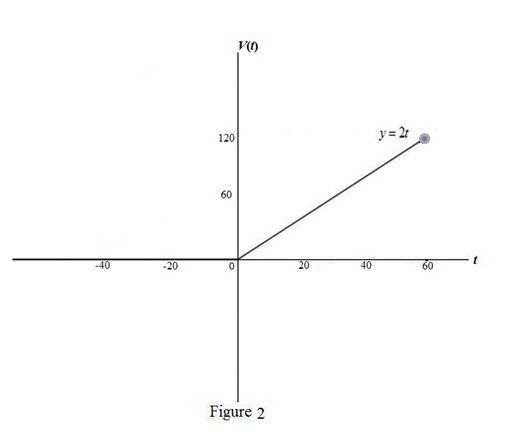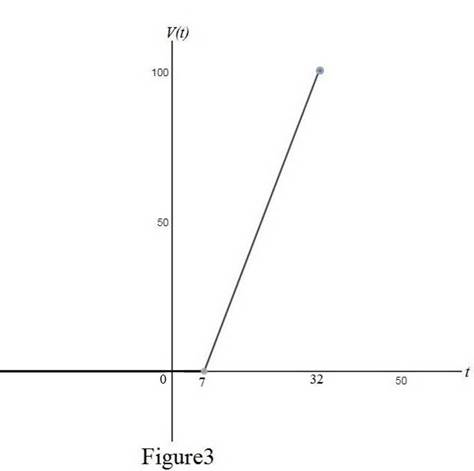# The graph of the ramp function y = t H ( t ) .### Single Variable Calculus: Concepts...

4th Edition
James Stewart
Publisher: Cengage Learning
ISBN: 9781337687805### Single Variable Calculus: Concepts...

4th Edition
James Stewart
Publisher: Cengage Learning
ISBN: 9781337687805

#### Solutions

Chapter 1.3, Problem 58E

(a)

To determine

## To sketch: The graph of the ramp function y=tH(t) .

Expert Solution

### Explanation of Solution

Heaviside function is, H(t)={0      if t<01if t0 .

So, the ramp function is, R(t)=tH(t)={0      if t<0tif t0 .

Use online graph calculator and draw the graph of the ramp function as shown below in Figure 1.From Figure1, it is noted that the graph is a ray with starting point 0.

(b)

To determine

### To sketch: The graph of circuit if the switch is turned at t = 0 and the voltage gradually increased to 120 volts in 60 seconds; write the formula for V(t) in terms of H(t) fort≤60 .

Expert Solution

The formula for V(t) in terms of H(t) is V(t)=2tH(t),t60 .

### Explanation of Solution

Given:

The voltage V(t) switched in time t=0 and 120 volts are gradually increased over a period t60 .

Calculation:

Let the ramp function be y=V(t)=ctH(t) .

Find the value of c.

Since t=60 , then the ramp function becomes, V(60)=c(60)H(t) (1)

It is known that H(t)=1 .

Therefore, equation(1) becomes,

120=c(60)(1)c=12060c=2

Thus, the ramp function is y=V(t)=2tH(t) .

Therefore, V(t) can be written in form of H(t) as, 2tH(t)={0        if t<02tif t0 .

Use online graph calculator and draw the graph of V(t)={0        if t<02tif t0 as shown below in Figure 2.From Figure1, it is noted that the graph is a ray with starting point 0.

(c)

To determine

### To sketch: The graph of the voltage in a circuit if the switch is turned at t = 7 seconds and gradually increased t0 100 volts over a period of 25 seconds; write the formula for V(t) in terms of H(t) for t≤32 .

Expert Solution

The formula for V(t) in terms of H(t) after t=7 is V(t)=4(t7)H(t7),t32

### Explanation of Solution

The voltage varies from 0 to 100 in 25 seconds.

Therefore,

c=100025=10025=4

Therefore, the ramp function becomes, V(t)=4tH(t)

The switch is turned at t = 7 seconds

That is, the time t7=0

Therefore, the formula for V(t) in terms of H(t) after t=7 is,. V(t)=4(t7)H(t7) .

Thus, V(t) is transferred to the 7 units to the right of the ramp function.

The function, V(t) can be written as, V(t)={0                    if t<74(t7)   if 7t32 .

Use online graph calculator and draw the graph of V(t)={0                    if t<74(t7)   if 7t32 as shown below in Figure 3.From Figure 3, it is noted that the voltage reaches to 100 in 25 seconds.

### Have a homework question?

Subscribe to bartleby learn! Ask subject matter experts 30 homework questions each month. Plus, you’ll have access to millions of step-by-step textbook answers!